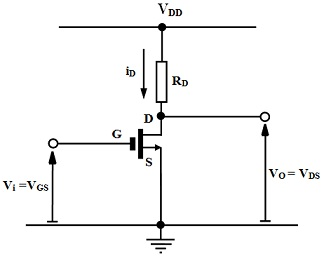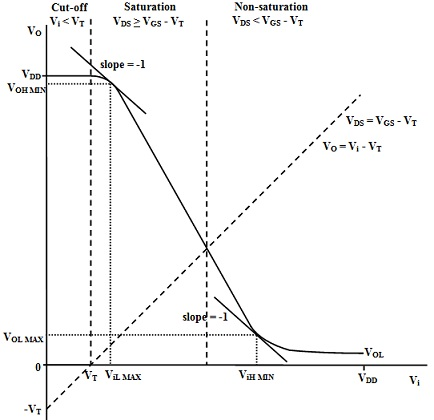#### Theory of Static Characteristics II of MOS Transistor Inverter

MOS Transistor Inverter: Static Characteristics II

MOS Inverter Voltage Transfer Characteristic:

The schematic figure of simple MOS transistor inverter with a resistive load is repeated in figure shown below. Since with the simple bipolar transistor inverter, the transfer characteristic can be plotted as output voltage against input voltage, Vo vs. Vin as shown in figure below.Figure: Schematic Diagram of Simple MOS Inverter

Initially, with Vi = 0 the input voltage to transistor is beneath threshold voltage and the transistor is OFF or non-conducting and therefore the output voltage is pulled up to the supply voltage VDD. Once the input voltage is raised to be equivalent to the threshold voltage, VT, the transistor starts to conduct and therefore the output voltage drops. As VDS > VGS – VT, the transistor operates initially in saturation region. Since the input voltage is further raised, the output voltage continues to drop until ultimately VDS < VGS – VT and the transistor comes out of the saturation region to operate in non-saturation region. Ultimately the input voltage reaches an utmost of VDD and the output reaches its minimum value of VOL as formerly computed.Figure: Voltage Transfer Characteristic of Simple MOS Transistor Inverter

Critical Logic Voltages:

The similar critical input and output logic voltages can be stated as for other logic families viz.:

ViLMAX = maximum voltage acceptable as the logic LO input

ViHMIN = minimum voltage acceptable as the logic HI input.

VOLMAX = maximum voltage acceptable as the logic LO output.

VOHMIN = minimum voltage acceptable as the logic HI output.

a) Critical Point ViL MAX, VOH MIN:

This is the point on upper left-hand portion of transfer characteristic where the slope is -1. At this point, the transistor can be taken to operate in the saturation region where, neglecting the consequences of channel length modulation for simplicity, the drain current is explained as:

ID = Kn(VGS - VT)2

However as VO = VDS and Vi = VGS and VO = VDD – iDRD then:

Vo = VDD – KnRD (Vi - VT)2.......................... (a)

On expanding it gives:

Vo = VDD - KnRDVi2 + 2 KnRDViVT - KnRDVT2

On differentiating:

∂Vo/∂Vi = - 2 KnRDVi + 2 KnRDVT

At critical point ∂Vo/∂Vi = -1 with Vi = ViL MAX and VO = VOH MIN and hence:

- 2 KnRDVi + 2 KnRDVT = - 1

2 KnRDViLMAX = 1 + 2 KnRDVT

And hence,

ViLMAX = VT + 1/(2 KnRD)

This value is a slight higher than VT and for illustration given with VT = 1V, RD = 100kΩ and Kn = 100µAV-2, ViL MAX = 1.05V.

Replacing back into equation (a) to find out the output voltage for this coordinate gives:

VOHMIN = VDD – KnRD(ViL MAX - VT)2

VOHMIN = VDD – KnRD[VT + (1/ KnRD) - VT]2

And hence ultimately:

VOHMIN = VDD – (1/4 KnRD)

This value is a slight lower than VDD and for illustration given with VDD = 10V, VT = 1V, RD = 100kΩ and Kn = 100µAV-2, VOH MIN = 9.98V. The coordinate of critical point (a) is then:

ViLMAX, VOHMIN = 1.05, 9.98 V

b) Critical Point ViH MIN, VOL MAX

This is the point on lower right-hand portion of the characteristic where slope is -1. At this point, the transistor can be taken to operate in non-saturation region where the drain current is explained as:

ID = Kn[2(VGS - VT)VDS – V2DS]

However again, as VO = VDS and Vi = VGS and VO = VDD – iDRD then:

VO = VDD – 2 KnRD (Vi - VT) Vo + KnRDVo2

On expanding:

VO = VDD – 2 KnRDViVo + 2 KnRDVTVo + KnRDVo2

On rearranging:

VO [1 - KnRDVT] = VDD - 2 KnRDViVo + KnRDVo2

There is a choice here to employ implicit differentiation to find ∂Vo/∂Vi or to re-arrange the expression as Vi in terms of VO and then determine ∂Vi/∂Vo. The latter is simpler as there is just one term in Vi. Then,

2 KnRDViVo = VDD – [1 - 2 KnRDVT]Vo + KnRDVo2

And hence,

Vi = VDD/(2 KnRDVo) – [(1 - 2 KnRDVT)/ 2 KnRD) + (Vo/2)............... (b)

Then,

∂Vo/∂Vi = - VDD/(2 KnRDVo2) + (1/2)

For ∂Vo/∂Vi  = -1 we can employ ∂Vi/∂Vo= -1 and hence:

- (VDD/2 KnRDVo2) + (1/2) = - 1

(VDD/2 KnRDVo2) = 3/2

Vo2 = VDD/3 KnRD

By taking the positive root as practical value gives:

VOLMAX = √VDD/3KnRD

That for the illustration given with VDD = 10V, VT = 1V, RD = 100kΩ and Kn = 100µAV-2 , VOL MAX = 0.58V.

This is significantly higher than the great value of VOL computed formerly. Then replacing this back into the expression for Vi in equation (b) above gives:And therefore ultimately the critical input value is as shown:

ViHMIN = VT + 2√VDD/3KnRD – (1/2 KnRD)

That for illustration given with VDD = 10V, VT = 1V, RD = 100kΩ and Kn = 100µAV-2 provides ViH MIN = 2.1V. This gives the coordinates of critical point (b) as:

ViHMIN, VOLMAX = 2.1, 0.58 V

Noise Margins:

Ultimately, the noise margins for simple MOS inverter can be computed approximately from the critical points evaluated from the transfer characteristic as shown:

NMH = VOHMIN – ViHMIN = 9.98 – 2.1 = 7.88V

NML = ViLMAX – VOLMAX = 1.05 – 0.58 = 0.47V

Latest technology based Electrical Engineering Online Tutoring Assistance

Tutors, at the www.tutorsglobe.com, take pledge to provide full satisfaction and assurance in Electrical Engineering help via online tutoring. Students are getting 100% satisfaction by online tutors across the globe. Here you can get homework help for Electrical Engineering, project ideas and tutorials. We provide email based Electrical Engineering help. You can join us to ask queries 24x7 with live, experienced and qualified online tutors specialized in Electrical Engineering. Through Online Tutoring, you would be able to complete your homework or assignments at your home. Tutors at the TutorsGlobe are committed to provide the best quality online tutoring assistance for Electrical Engineering Homework help and assignment help services. They use their experience, as they have solved thousands of the Electrical Engineering assignments, which may help you to solve your complex issues of Electrical Engineering. TutorsGlobe assure for the best quality compliance to your homework. Compromise with quality is not in our dictionary. If we feel that we are not able to provide the homework help as per the deadline or given instruction by the student, we refund the money of the student without any delay.